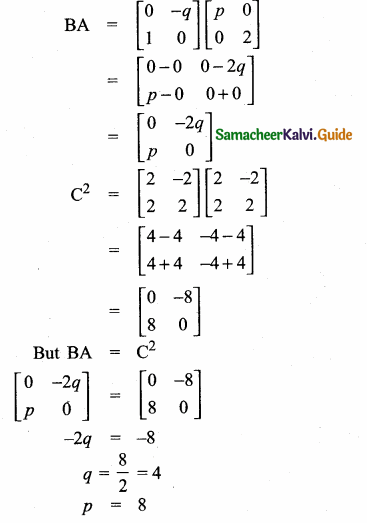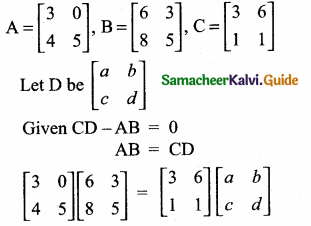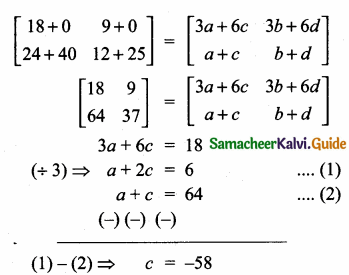Students can download Maths Chapter 3 Algebra Unit Exercise 3 Questions and Answers, Notes, Samacheer Kalvi 10th Maths Guide Pdf helps you to revise the complete Tamilnadu State Board New Syllabus, helps students complete homework assignments and to score high marks in board exams.

## Tamilnadu Samacheer Kalvi 10th Maths Solutions Chapter 3 Algebra Unit Exercise 3

Question 1.
Solve
$$\frac { 1 }{ 3 }$$ (x + y – 5) = y – z = 2x – 11 = 9 – (x + 2z)
$$\frac { 1 }{ 3 }$$ (x + y – 5) = y – z
x + y – 5 = 3y – 3z
x + y – 3y + 3z = 5
x – 2y + 3z = 5 ….(1)
y – z – 2x – 11
-2 x + y – z = -11
2x – y + z = 11 …..(2)
2x – 11 = 9-(x + 2 z)
2x – 11 = 9 – x – 2z
2x + x + 2z = 9 + 11
3x + 2z = 20 ….(3)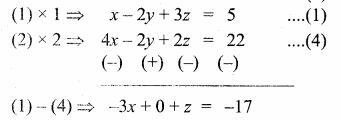3x – z = 17 …. (5)Substitute the value of z = 1 in (3)
3x + 2(1) = 20
3x = 20 – 2
3x = 18
x = $$\frac { 18 }{ 3 }$$ = 6
substitute the value of x = 6, z = 1 in (2)
2(6) – y + 1 = 11
12 – y + 1 = 11
13 – y = 11
-y = 11 – 13
-y = -2
y = 2
∴ The value of x = 6, y = 2 and z = 1Question 2.
One hundred and fifty students are admitted to a school. They are distrbuted over three sections A, B and C. If 6 students are shifted from section A to section C, the sections will have equal number of students. If 4 times of students of section C exceeds the number of students of section A by the number of students in section B, find the number of students in the three sections.
Let the number of students in section A be “x”
Let the number of students in section B be “y”
Let the number of students in section C be “z”
By the given first condition
x + y + z = 150 ……(1)
again by the second condition
x – 6 = z + 6
x – z = 6 + 6
x – z = 12 ….(2)
again by the third condition
x + y = 4z
x + y – 4z = 0
x + y – 4z = 0 ….(3)
Subtracting (1) and (3)Substitute the value of z = 30 in (2)
x – 30 = 12
x = 12 + 30
= 42
Substitute the value of x = 42 and z = 30 in (1)
42 + y + 30 = 150
y + 72 = 150
y = 150 – 72
= 78
Number of students in section A, B and C are = 42, 78 and 30.Question 3.
In a three-digit number, when the tens and the hundreds digit are interchanged the new number is 54 more than three times the original number. If 198 is added to the number, the digits are reversed. The tens digit exceeds the hundreds digit by twice as that of the tens digit exceeds the unit digit. Find the original number.
Let the hundred digit be x
the tens digit be y and the unit digit be z
∴ The number is 100x + 10y + z
By the given first condition
100y + 10x + z = 54 + 3 (100x + 10y + z)
100y + 10x + z = 54 + 300x + 30y + 3z
-290x + 70y – 2z = 54 (÷ -2)
145x-35y + z = -27 ….(1)
Again by the second condition
198 + 100x + 10y + z = 100z + 10y + x
99x – 99z = -198 (÷ 99)
x – z = -2 ….(2)
Again by the third condition
y – x = 2(y – z)
y – x = 2y – 2z
– x – y + 2z = 0
x + y – 2z = 0 ….(3)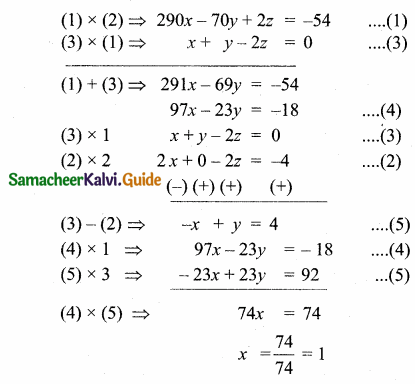substitute the value of x = 1 in …….(2)
1 – z = -2
3 = z
∴ z = 3
substitute the value of x = 1 and z = 3 in …….(3)
1 – y – 6 = 0
y – 5 = 0
y = 5
∴ The number is 153Question 4.
Find the least common multiple of xy(k2 +1) + k(x2 + y2) and xy(k2 – 1) + k(x2 – y2).
Solution:
xy (k2 + 1) + k (x2 + y2) …………… (1)
xy(k2 – 1) + k(x2 – y2) …………… (2)
(1) ⇒ xyk2 + xy + kx2 + ky2
(2) ⇒ xyk2 – xy + kx2 – ky2
(1) ⇒ yk (xk + y) + x (xk + y)
= (xk + y) (x + yk)
(2) ⇒ yk (xk – y) + x (xk – y)
= (x + yk) (xk – y)
∴ L.C.M. : (x + yk) (xk + y) (xk – y)
= (x + yk) (x2k2 – y2)Question 5.
Find the GCD of the following by division algorithm
2x4 + 13x3 + 27x2 + 23x + 7,
x3 + 3x2 + 3x + 1, x2 + 2x + 1
p(x) = 2x4 + 13x3 + 27x2 + 23x + 7
g(x) = x3 + 3x2 + 3x + 1
r(x) = x2 + 2x + 1
(i) Find the G.C.D. of p(x) and g(x)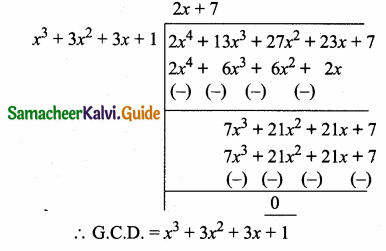(ii) Find the G.C.D. of r(x) and the G.C.D. of p(x) and g(x)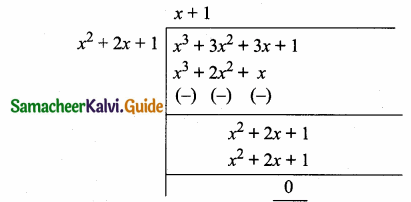∴ G.C.D.= x2 + 2x + 1
∴ G.C.D. of the three
polynomials = x2 + 2x + 1Question 6.
Reduce the given Rational expressions to its lowest form
(i) $$\frac{x^{3 a}-8}{x^{2 a}+2 x^{a}+4}$$
x3a – 8 = (xa)3 – 23
(using the formula a3 – b3 = (a – b)(a2 + ab + b2)
= (xa – 2)[(xa)2 + xa × 2 + 22]
= (xa – 2) (x2a + 2xa + 4)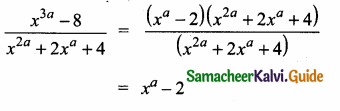(ii) $$\frac{10 x^{3}-25 x^{2}+4 x-10}{-4-10 x^{2}}$$
10x3 – 25x2 + 4x – 10 = 5x2(2x – 5) + 2 (2x – 5)
= (2x – 5) (5x2 + 2)
– 4 – 10x2 = -2 (2 + 5x2)
= -2(5x2 + 2)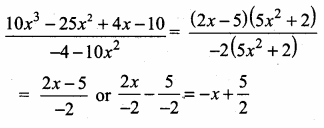Question 7.
Simplify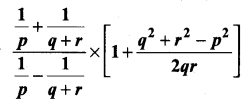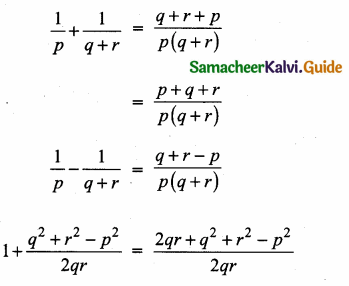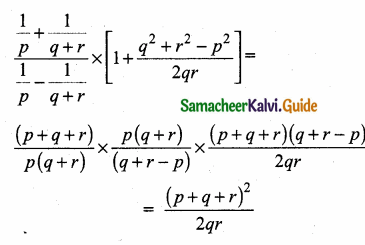Question 8.
Arul, Ravi and Ram working together can clean a store in 6 hours. Working alone, Ravi takes twice as long to clean the store as Arul does. Ram needs three times as long as Arul does. How long would it take each if they are working alone?
Let the time taken by Arul be “x” hours
Let the time taken by Ravi be “y” hours
Let the time taken by Ram be “z” hours
By the given first condition
$$\frac { 1 }{ x }$$ + $$\frac { 1 }{ y }$$ + $$\frac { 1 }{ z }$$ = $$\frac { 1 }{ 6 }$$
Again by the given second condition
$$\frac { 1 }{ x }$$ = 2 × $$\frac { 1 }{ y }$$
$$\frac { 1 }{ x }$$ – $$\frac { 2 }{ y }$$ = 0
By the given third condition
3 × $$\frac { 1 }{ z }$$ = $$\frac { 1 }{ x }$$
– $$\frac { 1 }{ x }$$ + $$\frac { 3 }{ z }$$ = 0
Let $$\frac { 1 }{ x }$$ = a, $$\frac { 1 }{ y }$$ = b, $$\frac { 1 }{ z }$$ = c
a + b + c = $$\frac { 1 }{ 6 }$$
6a + 6b + 6c = 1 …….(1)
a – 2b = 0 ……….(2)
-a + 3c = 0 …………(3)Arul take 11 hours, Ravi take 22 hours and Ram takes 33 hours.Question 9.
Find the square root of 289x4 – 612x3 + 970x2 – 684x + 361.Question 10.
Solve $$\sqrt { y+1 }$$ + $$\sqrt { 2y-5 }$$ = 3
$$\sqrt { y+1 }$$ + $$\sqrt { 2y-5 }$$ = 3
(squaring on bothsides)8y2 – 9y2 – 12y + 78y – 20 – 169 = 0
-y2 – 66y – 189 = 0
y2 – 66y + 189 = 0
(y – 3) (y – 63) = 0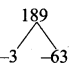y – 3 or y = 63
The value of y is 3 and 63Question 11.
A boat takes 1.6 hours longer to go 36 kins up a river than down the river. If the speed of the water current is 4 km per hr, what is the speed of the boat in still water?
Let the speed of the boat in still water be “x”
Time taken to go for up of a river = $$\frac { 36 }{ x+4 }$$
By the given condition
$$\frac { 36 }{ x-4 }$$ – $$\frac { 36 }{ x+4 }$$ = 1.6The speed of the boat in still water = 14 km/hrQuestion 12.
Is it possible to design a rectangular park of perimeter 320 m and area 4800 m2? If so find its length and breadth.
Let the length of the rectangular park be “l”
and the breadth of the rectangular park be “b”
Perimeter of the park = 320 m
2 (l + b) = 320
l + b = 160
l = 160 – b ……….(1)
Area of the park = 4800 m2
l × b = 4800 ….(2)
substitute the value of l = 160 – b in (2)
(160 – b)b = 4800
160b – b2 = 4800
b2 – 160b + 4800 = 0
(b – 120) (b – 40) = 0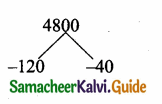b = -120 = 0 or b – 40 = 0
b = 120 or b = 40
If breadth is 120 length is 40
If breadth is 40 length is 120
Length of the park = 120 m
Breadth of the park = 40 mQuestion 13.
At t minutes past 2 pm, the time needed to 3 pm is 3 minutes less than $$\frac{t^{2}}{4}$$ Find t.
Time needed by the minutes hand to show
3 pm = (60 – 1) minutes
By the given condition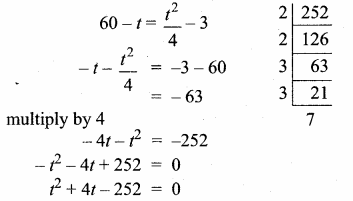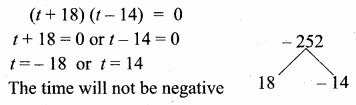∴ The value of t = 14 minutes

Question 14.
The number of seats in a row is equal to the total number of rows in a hall. The total number of seats in the hall will increase by 375 if the number of rows is doubled and the number of seats in each row is reduced by 5. Find the number of rows in the hall at the beginning.
Let the number of rows in the hall be “x”
∴ Total number of rows = x
Total number of seats in the hall is “x2”
By the given condition
x2 + 375 = 2x (x – 5)
x2 + 375 = 2x2– 10x
x2 – 2x2 + 10x + 375 = 0
– x2 + 10x + 375 = 0
– x2 – 10x – 375 = 0
(x – 25) (x + 15)
x – 25 = 0 or x + 15 = 0
x = 25 or x = – 15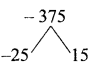Number of rows in the hall = 25Question 15.
If α and β are the roots of the polynomial f(x) – x2 – 2x + 3, find the polynomial whose roots are
(i) α + 2, β + 2
α and β are the roots of the polynomial
x2 – 2x + 3 = 0
α + β = 2; αβ = 3
(i) Sum of the roots = α + 2 + β + 2
= α + β + 4
= 2 + 4
= 6
Product of the roots = (α + 2) (β + 2)
= αβ + 2α + 2β + 4
= αβ + 2(α + β) + 4
= 3 + 4 + 4
= 11
x2 – (sum of the roots) x + product of the roots = 0
x2 – (6) x + 11 = 0
x2 – 6x + 11 = 0

(ii) $$\frac{\alpha-1}{\alpha+1}, \frac{\beta-1}{\beta+1}$$
Sum of the roots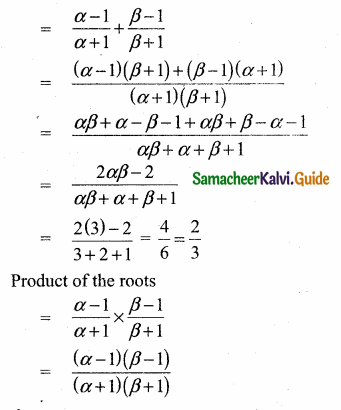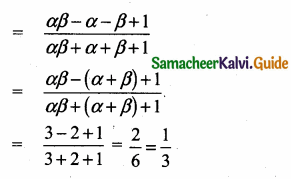x2 – (sum of the roots) + products of the roots = 0
x2 – ($$\frac { 2 }{ 3 }$$) x + $$\frac { 1 }{ 3 }$$ = 0
3x2 – 2x + 1 = 0Question 16.
If -4 is a root of the equation x2 + px – 4 = 0 and if the equation x2 + px + q = 0 has equal roots, find the values of p and q.
Solution:
f(x) = x2 + px – 4 = 0
If -4 is a root, then
f(-4) = (-4)2 + P(-4) – 4 = 16 – 4p – 4 = 0
12 – 4p = 0
-4p = -12
p = 3
x2 + 3x + q =0 has equal roots,
∆ = b2 – 4ac = 0
32 – 4 × 1 × q = 0
9 – 4q = 0
-4 q = -9
q = $$\frac{9}{4}$$
p = 3, q = $$\frac{9}{4}$$Question 17.
Two farmers Senthil and Ravi cultivates three varieties of grains namely rice, wheat and ragi. If the sale (in ₹) of three varieties of grains by both the farmers in the month of April is given by the matrix.and the May month sale (in ₹) is exactly twice as that of the April month sale for each variety.
(i) What is the average sales of the months April and May.
(i) Let A represent the sale on April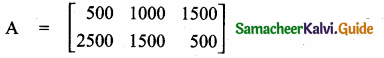Let B represent the sale on May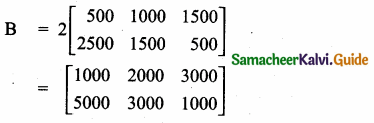Average sale of the month April and May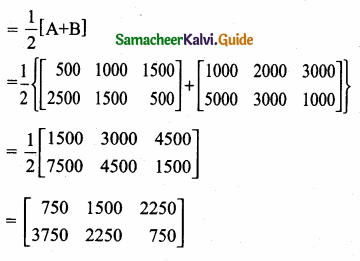(ii) If the sales continue to increase in the same way in the successive months, what will be sales in the month of August?
If it increasing in the successive months of
May sale is 2 (April sale)
June sale is 4 (April sale)
July sale is 8 (April sale)
August sale is 16 (April sale)
Sales in the month of AugustQuestion 18.
If cos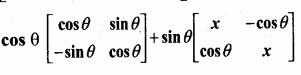= I2, find x.Question 19.
Givenand if BA = C2, find p and q